## 3.4.2 First-Order Perturbation Expansion

The electron-electron interaction up to the first-order perturbation is studied here. In the interaction representation the operator corresponding to this kind of interaction is given by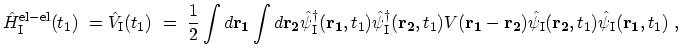(3.35)

where the COULOMB interaction potential is assumed to be an instantaneous potential proportional to a delta function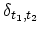. The first-order term of the perturbation expansion is given by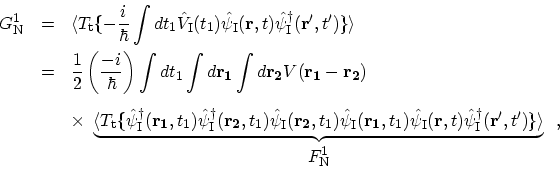(3.36)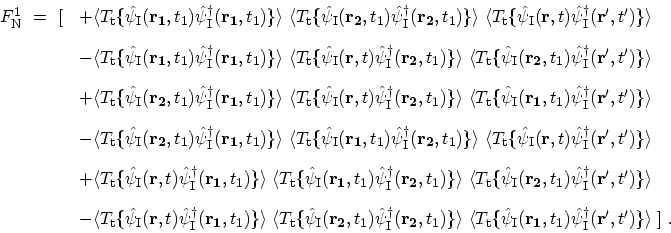(3.37)

By replacing the brackets by GREEN's functions one gets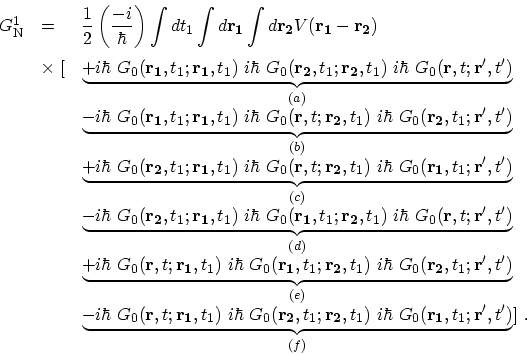(3.38)

Figure 3.4: FEYNMAN diagrams of the first-order perturbation terms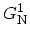.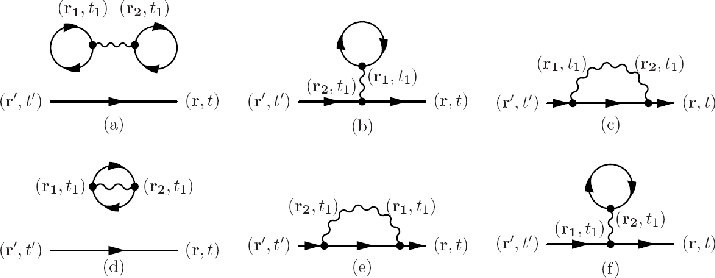FEYNMAN diagrams for the corresponding terms are shown in Fig. 3.4. In the first-order example the connected diagrams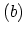and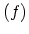are equal, as are the diagrams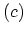and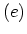; they differ only in that the integration variables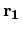and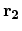are interchanged, whereas the COULOMB potential is symmetric under this substitution. It is therefore sufficient to retain just one diagram of each type, simultaneously omitting the factor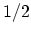in front of (3.38). For the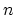th-order perturbation there are! possible interchanges of integration variables. Therefore, the repetition of the same diagrams cancels the factor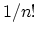in (3.31).

Diagram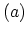and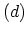contain sub-units that are not connected by any lines to the rest of the diagram. Feynman diagrams in which all parts are not connected are called disconnected diagrams. Equation (3.38) shows that such diagrams are typically have GREEN's function and interactions whose arguments close on themselves. As a result the contribution of this sub-unit can be factored out of the expression for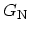. The same procedure can be applied for the denominator. In this cases, the second term of the expansion includes only two non-vanishing terms which are only disconnected diagrams of (3.38), namely (a) and (d).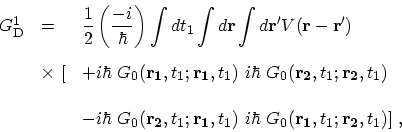(3.39)

As a result, these terms cancel the disconnected diagrams of the numerator and the resulting GREEN's function consists of only connected diagrams. It can be shown that in general the vacuum polarization terms cancel the disconnected diagrams in the expansion of the GREEN's function . As a result the GREEN's function is just the summation of all topologically different connected diagrams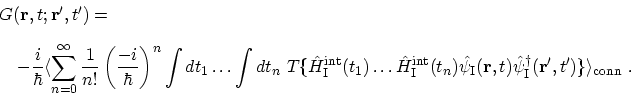(3.40)

M. Pourfath: Numerical Study of Quantum Transport in Carbon Nanotube-Based Transistors